: 4 Dust transport : Two dimensional anelastic model : 2 Atmospheric model

# 3 Turbulent parameterization

## 3.1 Subgrid turbulent mixing parameterization

The space differencing in the turbulent kinetic energy equation (equation (10) in Part I) is evaluated by the forth order centered scheme for advection terms and the second order centered scheme for other terms. In time integration, the forward scheme is adapted for the friction terms. Representations of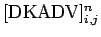and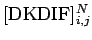are same as those of (22) and (24).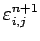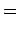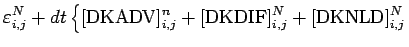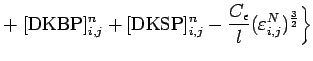(41)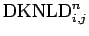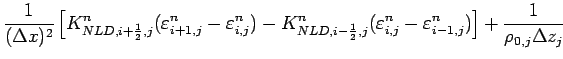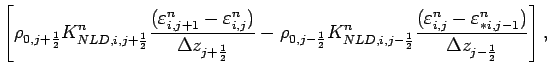(42)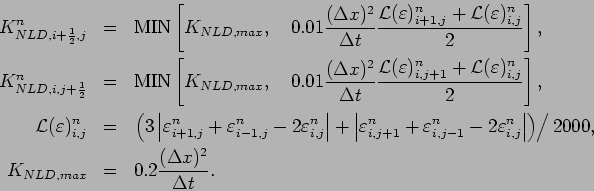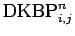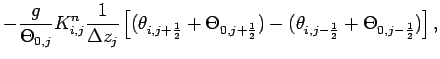(43)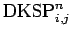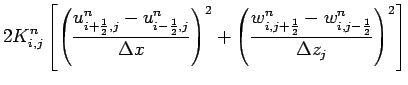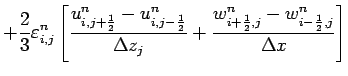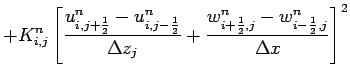(44)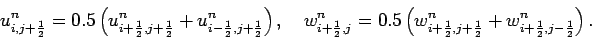## 3.2 Surface flux parameterization

The finite difference form of the surface flux are as follows.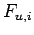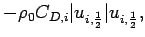(45)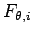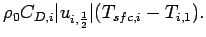(46)

where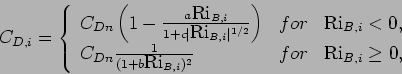(47)

The bulk Richardson number is calculated as follows.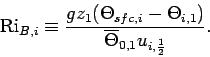(48): 4 Dust transport : Two dimensional anelastic model : 2 Atmospheric model
Odaka Masatsugu $BJ?@.(B19$BG/(B4$B7n(B26$BF|(B